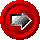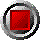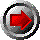Variable-coupon American & Bermudan Bond Options (HW-Tree)Variable-coupon American & Bermudan Bond Options (HW-Tree)

HWTree_BondAmerCallVar(argument list…)

HWTree_BondAmerPutVar(argument list…)

HWTree_BondBermCallVar(argument list…)

HWTree_BondBermPutVar(argument list…)

These functions return the values of American and Bermudan call and put options on variable coupon bonds with varying strike prices using the Hull-White trinomial tree interest rate model. The functions use the following arguments:

 Argument Description Restrictions Val_Date valuation date (e.g. today) valid Excel date number Bond_Settlement_Lag number of business days until settlement for bond transaction made on Valuation_Date >= 0 Bond_Maturity bond maturity date > Valuation_Date (with settlement lag) Freq number of bond coupons per annum 1, 2, 4, or 12 DCB day count basis 0 = 30/360 (US) 1 = act/act for CAD/US T-Bonds 2 = act/360 3 = act/365 4 = 30/360 (European) Coupon_Dates array of bond coupon dates First element must be the last coupon date before the settlement date, or an even older coupon, and last element must be the maturity date of the bond. Dates must be in ascending order, and consistent with the coupon frequency and maturity date. Coupon_Rates array of bond coupon rates, as annualized rates, in decimal form (e.g. six percent entered as 0.06) >= 0, and corresponding to the Coupon_Dates array Last_Exer_Date is the final option exercise date; is used to provide a final exercise date for American options (see Strike_Dates) < Bond_Maturity Notice_Days the number of days needed to give notice for option exercise >= 0 Strike_Dates an array of exercise dates for the option; for American options, it is the date the strike price changes (steps up/down) to the next strike rate (i.e. strike price is in effect from the strike date to the next one, or to the Last_Exer_Date ascending order Strike_Prices an array of option strikes per \$100 par, corresponding to the strike dates > 0 array must be same size as Strike_Dates Holidays array of holiday dates valid Excel date numbers strictly ascending order Short_Rate_Vol annual standard deviation of the short rate of interest, in decimal form > 0 Reversion_Rate mean reversion rate of the short rate of interest, in decimal form >= 0 Tree_Steps number of steps in trinomial tree BETWEEN THE NEXT AND FINAL EXERCISE DATES > 0, typically 50 to 100, although this should be lowered if the exercise period is a small fraction of the life of the option (otherwise too many tree steps in total) Zero_Dates array of zero coupon curve dates strictly ascending order The first date of this array must be Valuation_Date Zero_Rates array of continuously compounded riskless rates in decimal form corresponding to Zero_Dates > 0 OAS parallel shift of the zero curve in decimal form Bucket_Start start of bucket for zero curve shifts set to 0 if curve shift is not desired Bucket_End end of bucket for zero curve shift >= Bucket_Start Bucket_Shift parallel shift of the zero curve between Bucket_Start and Bucket_End in decimal form set to 0 if curve shock is not desired

© 1995-98 Leap of Faith Research Inc.